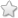# TNSB - 8 - Maths :: Numbers

## Course Content / Subtopic list:

1. Introduction To Square Numbers - Summing Up Odd Numbers From One Results In A Square, Summing Up Two Consecutive Natural Numbers Results In A Square, Finding The Square Of A Number Using Diagonal Method, Pythagorean Triplets
2. Square Root - Square Root By Repeated Subtraction Of Successive Odd Numbers; Square Root Through Prime Factorisation; Finding The Square Root Of A Number By Long Division Method; Number Of Digits In The Square Root Of A Perfect Square Number; Square Root Of Decimal Numbers; Square Root Of Product And Quotient Of Numbers; Approximating Square Roots.
3. Cubes And Cube Numbers - Properties, Cube Root, Cube Root Of A Given Number By Prime Factorisation
4. Exponents And Powers - Powers With Zero And Negative Exponents, Expanded Form Of Numbers Using Exponents, Laws Of Exponents, Standard Notation And Scientific Notation.

0.00(0 votes)